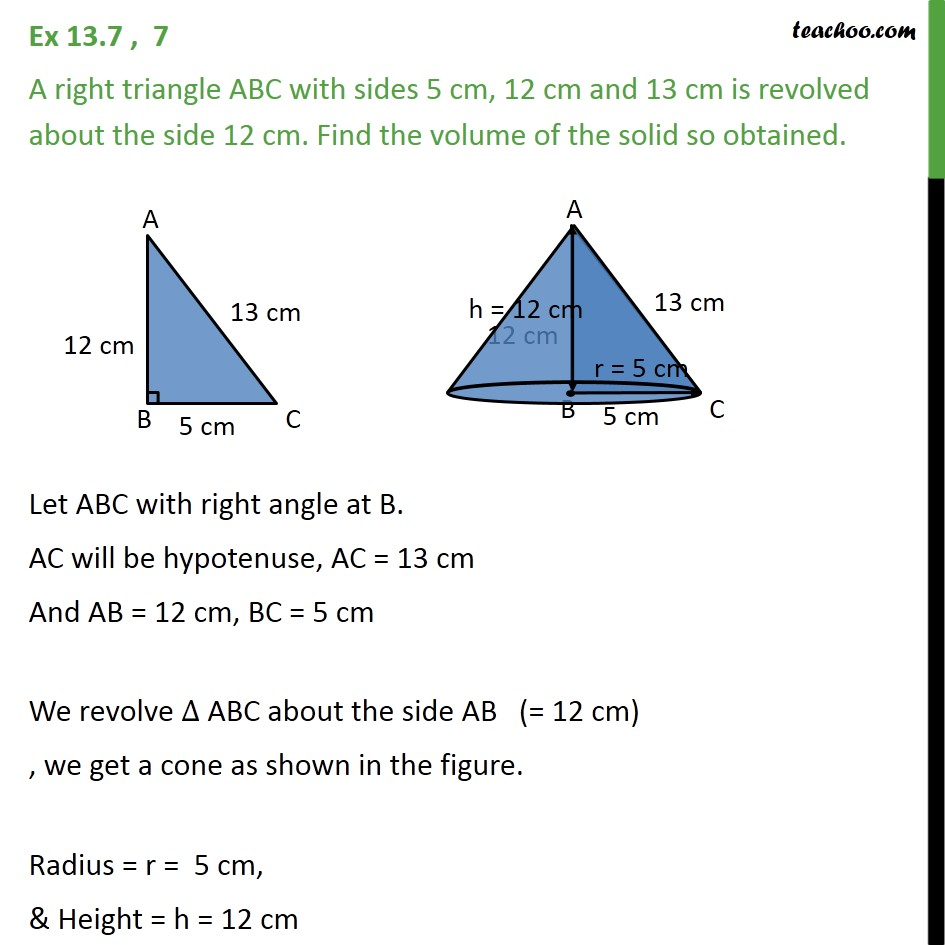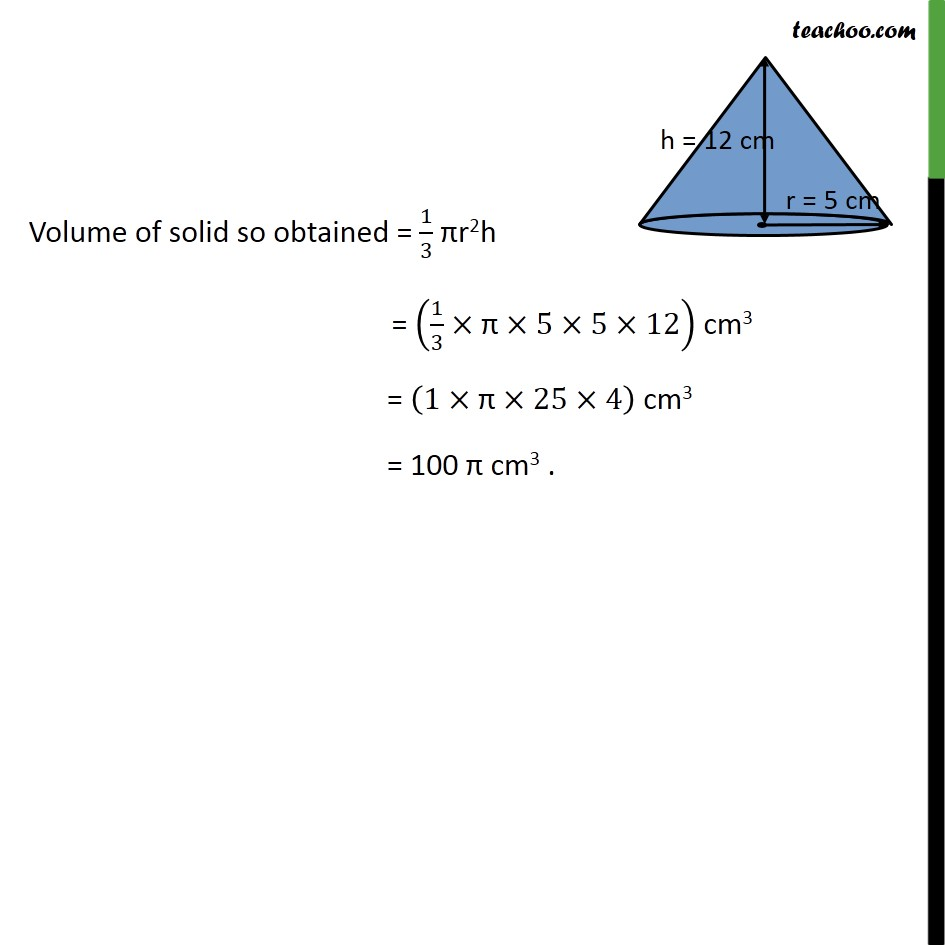Ex 11.3

Chapter 11 Class 9 Surface Areas and Volumes
Serial order wiseLearn in your speed, with individual attention - Teachoo Maths 1-on-1 Class

### Transcript

Ex 11.3 , 7 A right triangle ABC with sides 5 cm, 12 cm and 13 cm is revolved about the side 12 cm. Find the volume of the solid so obtained. Let ABC with right angle at B. AC will be hypotenuse, AC = 13 cm And AB = 12 cm, BC = 5 cm We revolve ABC about the side AB (= 12 cm) , we get a cone as shown in the figure. Radius = r = 5 cm, & Height = h = 12 cm Volume of solid so obtained = 1/3 r2h = (1/3 " " 5 5 12) cm3 = (1 " " 25 4) cm3 = 100 cm3 .